# Get the Sum of text values like numbers in Excel

In this article, we will learn how to Get the Sum of text values like numbers in Excel.

In simple words, while working with text values in dataset. Sometimes given a condition i.e. when we need to get the sum of the text values where a unique code is assigned to the text value. You can perform the solution to this problem easily using the excel functions as explained below.

How to solve the problem?

For this article we will be required to use the SUM function & INDEX function. Now we will make a formula out of these functions. Here we are given a range and list with code value. We need to find the SUM of the range of text values using its code from the list.

Generic formula:

{ = SUM ( INDEX ( code, N ( IF ( 1, MATCH ( range, list, 0) ) ) ) ) }

range : range of values where sum is calculated

list : list of text values along with its respective values.

code : numerical values corresponding to the list values

Example:

All of these might be confusing to understand. So, let's test this formula via running it on the example shown below.

Here we have a world table and each country is assigned with a code value and we need  to find the sum of the codes for its respective continent.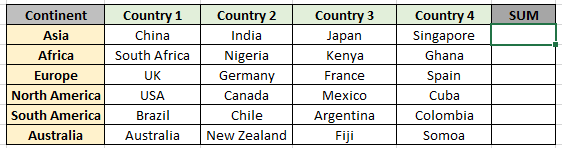List and code providing information to the above table is shown below.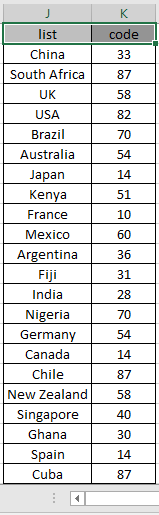Here the list & code range is given as using the named range excel tool.

Firstly, we need to find the values corresponding to the text values, extract numerical values and then sum operation be performed over those values. Now we will use the following formula to get the sum.
Use the Formula:

{ =SUM ( INDEX ( code, N ( IF ( 1, MATCH ( D3:G3 , list , 0 ) )) ) ) }

DONOT use curly braces manually. Curly braces applied using the Ctrl + Shift + Enter in place of just Enter.

Explanation:

• MATCH function returns the row index for the D3:G3 values from the exact values matched in the list values as shown below.

= SUM ( INDEX ( code , N ( IF ( 1 , { 1 , 13 , 7 , 19 } ) ) ) )

• N & IF function forces the formula to return array to the array as argument to the INDEX function.

= SUM ( INDEX ( code , { 1 , 13 , 7 , 19 } ) )

• Now INDEX function matches the ROW number in the code values and return corresponding code values to the SUM function.
• SUM function performs the sum operation over those values and returns the sum as shown below.
• This is an array formula as the array of values gets transferred in the formula.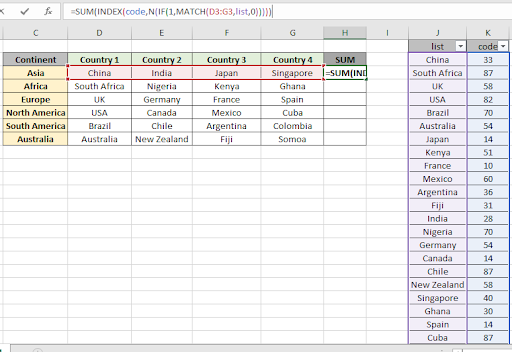Here the Code & lit range is given as the named range. Press Ctrl + Shift + Enter to get the SUM of the required text values as this is an array formula.As you can see in the above snapshot that sum of { 33 , 14 , 28 , 40 } comes out to be 115.

You can check the results by using the filter option on the list and code table as shown below. Apply the filter and select the required values.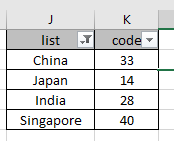Now copy the formula into other cells using the Ctrl + D or dragging down the cell option in excel.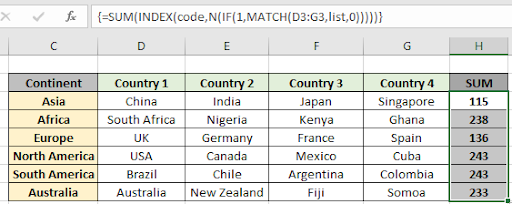As you can see in the above snapshot we obtained all the sum corresponding to the text values by extracting their code values.

Here are some observational notes shown below.

Notes:

1. The formula only works with numbers.
2. The formula works only when there are no duplicates in the list & code table.
3. The MATCH function takes 0 as an argument to get the exact match from the list.
4. DONOT use curly braces manually. Curly braces applied using the Ctrl + Shift + Enter in place of just Enter as it is an array formula.
5. The list array length must be of the same as the code length else the function.

Hope this article about how to Get the Sum of text values like numbers in Excel is explanatory. Find more articles on SUMPRODUCT functions here. Please share your query below in the comment box. We will assist you.

If you liked our blogs, share it with your friends on Facebook. And also you can follow us on Twitter and Facebook. We would love to hear from you, do let us know how we can improve, complement or innovate our work and make it better for you. Write us at info@exceltip.com

Related Articles

How to use the SUMPRODUCT function in Excel: Returns the SUM after multiplication of values in multiple arrays in excel.

SUM if date is between : Returns the SUM of values between given dates or period in excel.

Sum if date is greater than given date: Returns the SUM of values after the given date or period in excel.

2 Ways to Sum by Month in Excel: Returns the SUM of values within a given specific month in excel.

How to Sum Multiple Columns with Condition: Returns the SUM of values across multiple columns having condition in excel

How to use wildcards in excel : Count cells matching phrases using the wildcards in excel

Popular Articles

50 Excel Shortcut to Increase Your Productivity

Edit a dropdown list

Absolute reference in Excel

If with conditional formatting

If with wildcards

Vlookup by date

Convert Inches To Feet and Inches in Excel 2016

Join first and last name in excel

Count cells which match either A or B

Terms and Conditions of use

The applications/code on this site are distributed as is and without warranties or liability. In no event shall the owner of the copyrights, or the authors of the applications/code be liable for any loss of profit, any problems or any damage resulting from the use or evaluation of the applications/code.